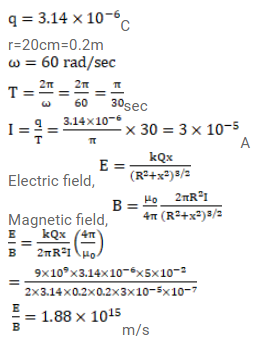# A charge of

Question:

A charge of $3.14 \times 10^{-6} \mathrm{C}$ is distributed uniformly over a circular ring of radius $20.0 \mathrm{~cm}$. The ring rotates about its axis with an angular velocity of $60.0 \mathrm{rad} / \mathrm{s}$. Find the ratio of the electric field to the magnetic field at a point on the axis at a distance of $5.00 \mathrm{~cm}$ from the center.

Solution: